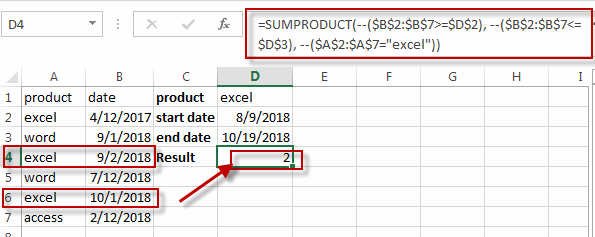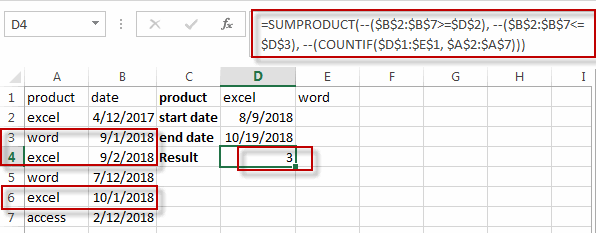Count Cells between Two Dates with Multiple Criteria

This post will guide you how to count cells between two dates with multiple criteria in excel. How do I count records between two dates and multiple criteria with formula in excel. You can easily count cells between two dates using COUNTIFS function. and if you want to count records between two dates with two or more criteria, how to achieve it.

Count Cells between Two Dates with Multiple Criteria

Assuming that you have a list of data that contain two columns, and one column contain product name and another column contain date values, and you want to count the number of product “excel” which is between two dates (from 2018/8/9-2018/10/19) in column B. You can create a new excel formula based on the SUMPRODUCT function to achieve the result.

=SUMPRODUCT(--(\$B\$2:\$B\$7>=\$D\$2), --(\$B\$2:\$B\$7<=\$D\$3), --(\$A\$2:\$A\$7="excel"))

Type this formula into a blank cell and then press Enter key in your keyboard.You will see that the count number will be shown.

If you want to count the number of the product “excel” and “word” which between two dates in column B, and you can refer to the above formula to write down another complex formula based on the SUMPRODUCT function and the COUNTIF function.

=SUMPRODUCT(--(\$B\$2:\$B\$7>=\$D\$2), --(\$B\$2:\$B\$7<=\$D\$3), --(COUNTIF(\$D\$1:\$E\$1, \$A\$2:\$A\$7)))

Type this formula into a blank cell and press Enter key. you will get the result.Related Functions

• Excel COUNTIF function
The Excel COUNTIF function will count the number of cells in a range that meet a given criteria. This function can be used to count the different kinds of cells with number, date, text values, blank, non-blanks, or containing specific characters.etc.= COUNTIF (range, criteria)…
• Excel SUMPRODUCT function
The Excel SUMPRODUCT function multiplies corresponding components in the given one or more arrays or ranges, and returns the sum of those products.The syntax of the SUMPRODUCT function is as below:= SUMPRODUCT (array1,[array2],…)…

Related Posts

Case Sensitive Lookup with SUMPRODUCT and EXACT

Today, we will show you how to use SUMPRODUCT and EXACT to perform a case sensitive exact match. In this article, we provide a simple example to calculate bonus for employees whose names are case-sensitive. If you meet similar scenarios ...

Average per Week by Formula in Excel

We usually apply AVERAGE function or relevant functions to return average directly in Excel worksheet. But in some situations, only applying average relevant functions cannot figure out our problem. Sometimes we can create a formula with functions and mathematical operation ...

How to Sum if Equal to Many Items or A Range in Excel

If we want to sum numbers from a range with criteria, we often select SUMIF of SUMIFS functions as the first choice to create a formula. The criteria can be a number or an array or a collection of some ...

How to Sum if Equal to X or Y in Excel

In daily work, if we want to sum numbers from a range, and only sum the numbers which being equal to X or Y in the range, we can create a formula with Excel build-in functions to get the result. ...

How To Sum the Largest N Values in Excel

Sometimes we may want to sum the largest N numbers or top N numbers in a range. In this article, we will show you the method of “SUM the Largest N Numbers” by a simple formula which consist of SUMPRODUCT ...

How to Sum for Cell Contains Formula Only in Excel

Sometimes values are created by formulas in cells. If we want to sum values which are created by formulas from a range, but some values which are hardcoded also list in the same range, how can we filter out matched ...

How to Sum the Smallest N Values in Excel

Sometimes we may want to sum the first smallest N numbers in a range in Excel. In this article, we will show you the method of “SUM the Smallest N Numbers” by a simple formula which consist of SUMPRODUCT and ...

How to Sum by SUMPRDUCT with One Specific Criteria Multiple Columns in Excel

Sometimes we may meet the case that to sum numbers based on one specific criteria. In this article, we will show you the method to resolve this problem by formula with the help of Excel SUMPRODUCT function. SUMPRODUCT can filter ...

How to Sum by SUMPRDUCT with Specific Criteria in Excel

Sometimes we may meet the case that to sum numbers based on two or more specific criteria. In this article, we will show you the method to resolve this problem by formulas with the help of Excel SUMPRODUCT function. SUMPRODUCT ...

How to Count Unique Numeric Values in a Range in Excel

This post will guide you how to count unique numeric values in the given range in Excel 2013/2016 or Excel office 365. How do I count the unique numeric values in a list of data with some duplicate values using ...

Sidebar